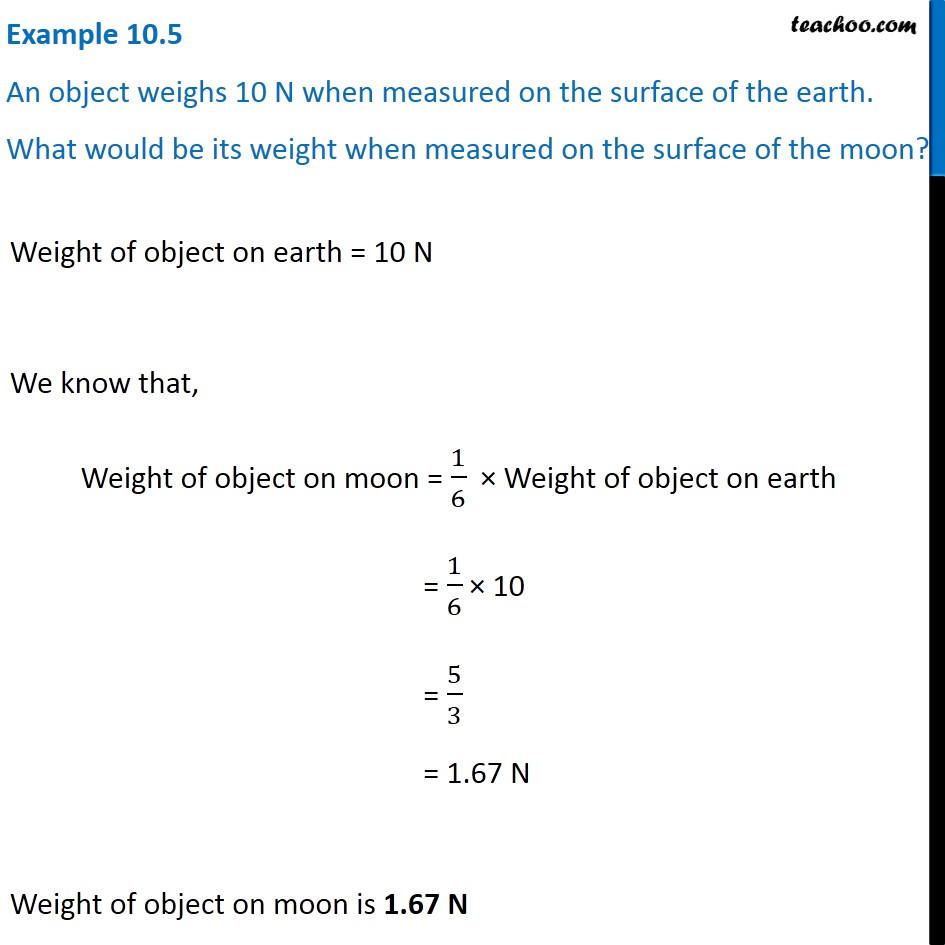Examples from NCERT Book

Class 9
Chapter 10 Class 9 - GravitationLearn in your speed, with individual attention - Teachoo Maths 1-on-1 Class

### Transcript

Example 10.5 An object weighs 10 N when measured on the surface of the earth. What would be its weight when measured on the surface of the moon? Weight of object on earth = 10 N We know that, Weight of object on moon = 1/6 × Weight of object on earth = 1/6 × 10 = 5/3 = 1.67 N Weight of object on moon is 1.67 N